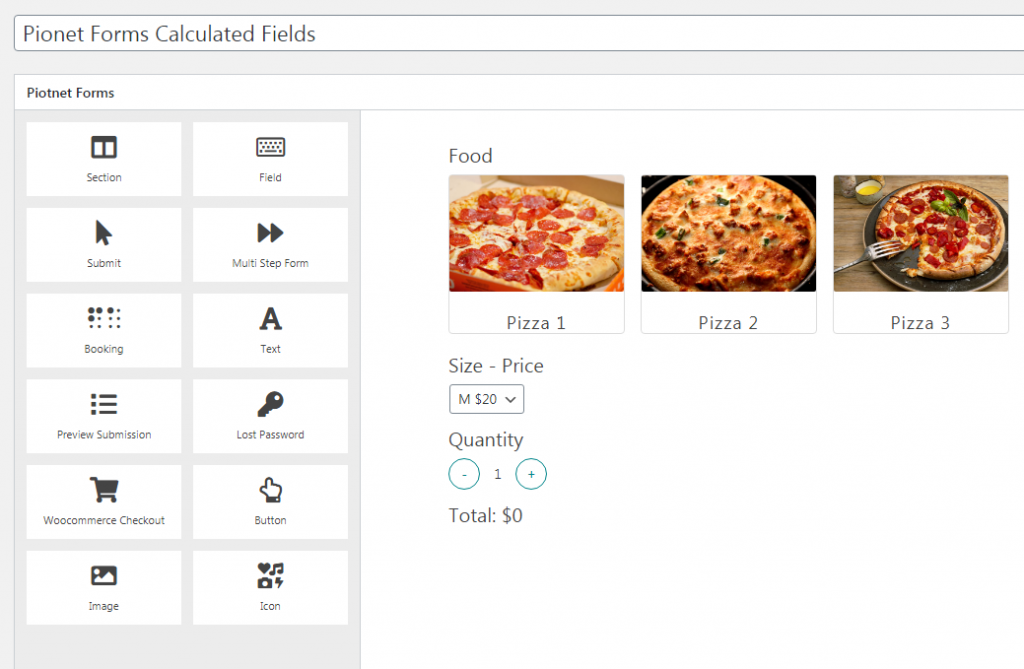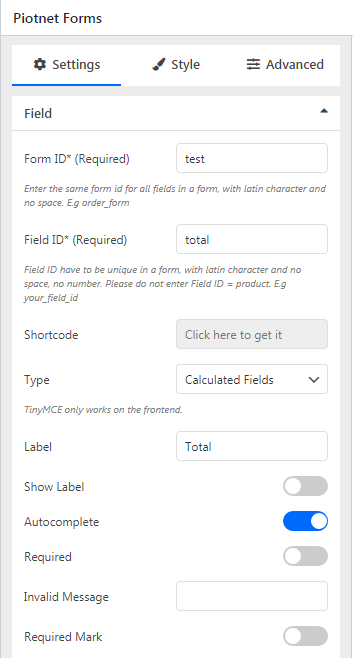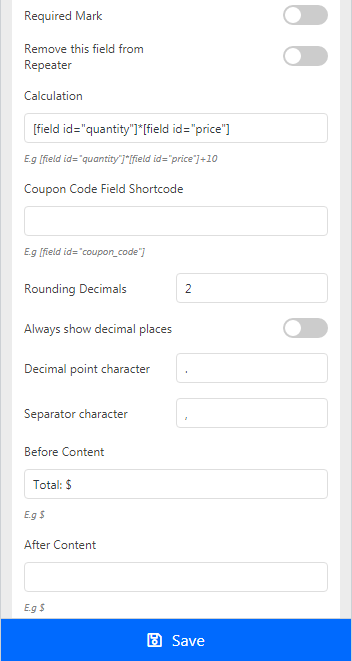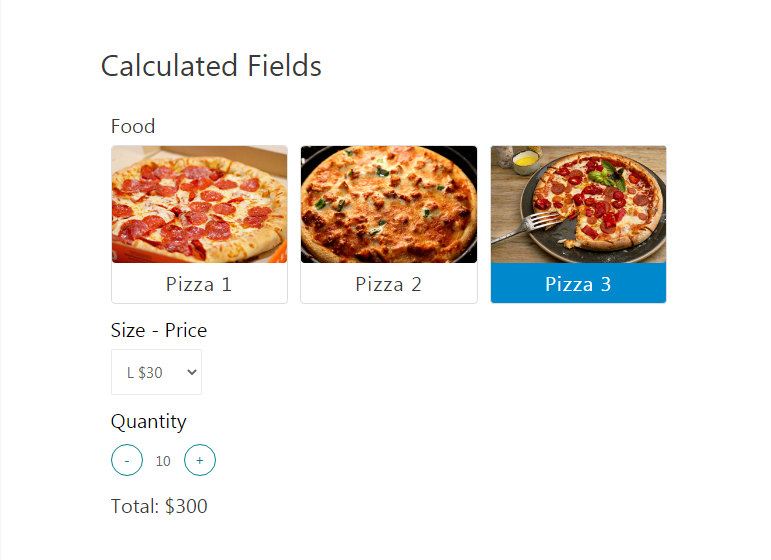HIDE
Basic Features

# Calculated Fields

By Calculated Fields, you can create calculations in your form quickly and conveniently. The plugin supports various mathematic operators for any of your calculating needs.

Example: Below is a procedure how to creat a bill calculation.

Firstly, we create a simple form which allow customers to choose product, size and quantity.Set Total field to Calculated type, the control section would be showed as below:In the Calculation control field, add your mathematic fomula. Shortcode of fields would be used as variables.

After choose product, size and quantity, Calculated Fields will automatically calculated total amount.